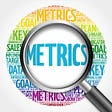# Let’s Explore Evaluation metrics for Classification and Regression

Water, water everywhere and all the boards did shrink,

Water water everywhere but nor any drop to drink

Time for a fun activity!

Let’s change some words in the above lines 💡

“ML, ML everywhere and all other technologies got replaced,

ML, ML everywhere, ‘from sklearn.import__’, just cut, copy and paste”

Sounds funny, Isn’t it?

Well, no doubts that Machine learning today has become one of the most quintessential Technologies without which it’s even becoming impossible for us to imagine our world. But before diving deep into this web we should know some of the basic yet important aspects of Machine Learning. One of which involves the use of correct evaluation metrics.

Want to Boost your model performance?

Let’s explore EVALUATION METRICS

Evaluation metrics are the measures on the basis of which we check how our model is performing. Just building a predictive model is not our motive.

A good analyst will always lookout for high accuracy on unseen data. After all, real-life projects need more data analysis than building the models.

Haha! Don’t get worried, this article describes evaluation metrics for Regression in detail so that you never have to worry about your model performance.

## 1. MSE: Mean Squared Error

Though its name in itself says it's mean in nature, but don’t worry, it’s going to help you a lot.

Okay, let’s now understand what MSE is:

“In Statistics, the mean squared error (MSE) or mean squared deviation (MSD) of an estimator (of a procedure for estimating an unobserved quantity) measures the average of the squares of the errors — that is, the average squared difference between the estimated values and the actual value.”

In simple words, it is the squared difference between the actual value and predicted value divided by the total number of observations.

Now that we know what MSE is, let’s understand how it helps in the optimization of our model?

MSE gives us an idea of how much our predictions variate from the actual results. The lower the MSE, the good is our model. Though it is a good measure to be considered but in cases where we do not perform scaling on either our training features or target features, it could be a large value depicting a bad model. In place of MSE, we can use RMSE which is the square root of MSE and gives us a better idea of model performance.

MSE can be calculated in Python using sklearn package.

from sklearn.metrics import mean_squared_error

import math

print(mean_squared_error(Y_test, Y_predicted))

print(math.sqrt(mean_squared_error(Y_test, Y_predicted)))

## 2. MAE: Mean Absolute Error

MAE is similar to MSE, just instead of squaring the error, we take the absolute difference between the predicted value and actual value divided by the total number of observations.

Now, let me ask you a question. If you have worked with Linear regression then you must know that in cost function, we take the square of difference and not the absolute difference, even after knowing that squaring the difference may result in large value. Give a minute and think about it.

Now let me tell you a secret!

The time when Linear Regression Algorithm was developed, mathematicians did not know how to find the derivative of modulus function (consider MAE). And since gradient descent required finding derivative of cost function (to find the minima), the MAE could not be used then. But today you can use either MAE formula or MSE formula in cost function to determine the minima.

from sklearn.metrics import mean_absolute_error

print(mean_absolute_error(Y_test, Y_predicted))

## 3. R- Square

R-square is considered the most effective metric for a Regression Model. If stated simply, R-Square is the square of the correlation coefficient ‘r’, whose value always lie between 0 and 1. While ‘r’ explains the relation between 2 variables (independent and dependent), r-square explains the proportion of variance for a dependent variable that’s explained by an independent variable or variables.

Let’s say the value of r-square is 0.6, it explains that more than 50% of the variation is explained by the independent feature.

R-square is calculated using the following formula:

## A large r-square value denotes a good fit of regression model.

However, r-square may fail in cases of overfitting. Because there may be many independent features which will make model fit the best on training data but fails badly on test data. To remove this problem, we use adjusted r-square instead of r-square. Adjusted r-square takes into account all the independent features and give the output on that basis.

from sklearn.metrics import r2_score

print(r2_score(y_true, y_pred))

## CONCLUSION

Do not blindly believe on just one metric and think your model performance is good. It’s always better to take into account adjusted r-square and MSE to see how the model performs on unseen data. And even before making any model and checking for its performance, always observe your data first and do a thorough Data Analysis. If its done perfectly, then believe that half of your problem is solved. All the best!

--

--

--

## More from DSC Banasthali Vidyapith

Psst…the last HINT here🎈 : “Follow our handle at one of the coming plots. The plot is a nest to a bird with amazing spaces, communities and thoughts”🐦

Love podcasts or audiobooks? Learn on the go with our new app.

## Applying Machine Learning on Diabetes Dataset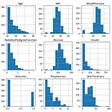## The Myth of the Impartial Machine## 7 Best Machine Learning and Deep Learning Courses## Advancing Machine Learning In Car Insurance## Learning Day 47: Computer vision before deep learning 1 — Image segmentation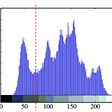## Understanding BERT — Part 1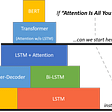## Seismic data analysis using AI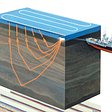## Machine Learning in Medicine — Part III## DSC Banasthali Vidyapith

Psst…the last HINT here🎈 : “Follow our handle at one of the coming plots. The plot is a nest to a bird with amazing spaces, communities and thoughts”🐦

## Randomized Optimization Algorithm Comparison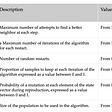## Dataset Selection## Data Discretization## Epitomes of Evaluation Metrics